Courses

# Shear Force & Bending Moment Notes | EduRev

## Civil Engineering (CE) : Shear Force & Bending Moment Notes | EduRev

The document Shear Force & Bending Moment Notes | EduRev is a part of the Civil Engineering (CE) Course Solid Mechanics.
All you need of Civil Engineering (CE) at this link: Civil Engineering (CE)

Shear Force and Bending Moment Diagrams

Beam is one of the most important structural components. Beams are usually long, straight, prismatic members and always subjected forces perpendicular to the axis of the beam

• A Shear Force Diagram (SFD) indicates how a force applied perpendicular to the axis (i.e., parallel to cross-section) of a beam is transmitted along the length of that beam.
• A Bending Moment Diagram (BMD) will show how the applied loads to a beam create a moment variation along the length of the beam.

Types of Supports

1. Roller Support: Resists vertical forces only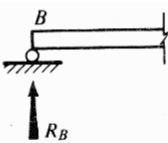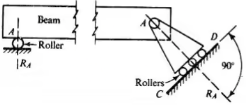2. Hinge support or pin connection: Resists horizontal and vertical forces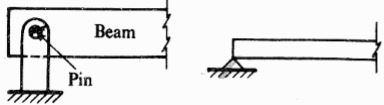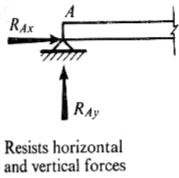Hinge and roller supports are called as simple supports
3. Fixed support or built-in end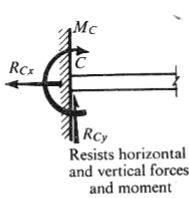The distance between two supports is known as “span”.

Types of beams

Beams are classified based on the type of supports:

1. Simply supported beam: A beam with two simple supports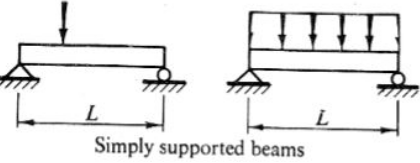2. Cantilever beam: Beam fixed at one end and free at other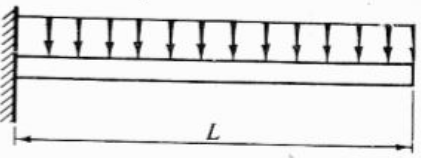3. Overhanging beam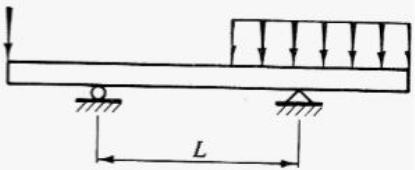4. Continuous beam: More than two supports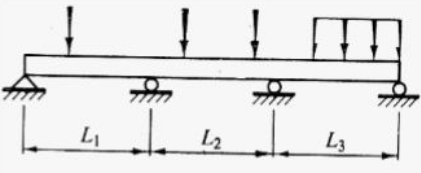Shear Force
Shear force has a tendency to slide the surface, it acts parallel to surface.
∑Fvert = 0
V - qdx - (V+dV) = 0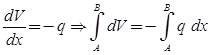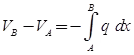Bending Moment
Any moment produced by forces acting on the beam must be balance by an equal opposite moment produced by internal forces acting in beam at the section. This moment is called bending moment.
∑M = 0
-M - qdx(dx / z) - (V + dV)dx + M + dm = 0
dM/dx = V ⇒ Ma - MA = ∫V dx
Only for distributed and concentrated load not for couple.
The necessary internal forces to keep the segment of the beam in equilibrium are
∑Fx = 0 ⇒ P
∑Fy = 0 ⇒ V
∑Fz = 0 ⇒ M

Differential equations of equilibrium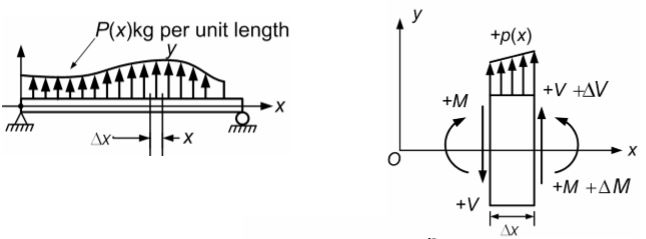Sign Conventions:
[∑Fx = 0 → +]
[∑Fy = 0 ↑ +]
So the differential equations would be: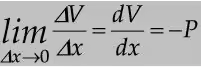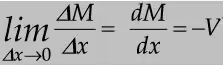From equation dV/dx = -P, we can write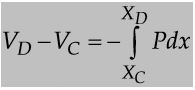From equation dy/dx = -M, we can write
MD - MC = -∫Vdx

Statically Determinate Beam
A beam is said to be statically determinate if all its reaction components can be calculated by applying three conditions of static equilibrium i.e.,

∑ V = 0, ∑ H = 0 and ∑ M = 0

Statically Indeterminate Beam
When the number of unknown reaction components exceeds the static conditions of equilibrium, the beam is said to be statically indeterminate.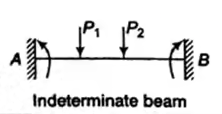Offer running on EduRev: Apply code STAYHOME200 to get INR 200 off on our premium plan EduRev Infinity!

## Solid Mechanics

31 videos|27 docs|29 tests

,

,

,

,

,

,

,

,

,

,

,

,

,

,

,

,

,

,

,

,

,

;